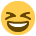# PEV June 08 ratios

Just a quickie (although it's way too late now for worrying) :001_smile:

Certain ratios, on a college handout we were given states to use the net profit, so does it matter if some use the net and some use the operating profit? I know that sometimes the AAT will mark both right (as seen in some past papers).

OMG, That PEV paper was just a nightmare from start to finish anyway, the wording and the questions were so weird.

Fingers crossed for August eh!

Bex

xx

• When it asked for the net profit margin I would assume it wanted the net profit used...thats my thinking :glare:
• Yes I would do the same, use the Net Profit instead of the Operating Profit
• Hi Michaela / Laura,

That's what I thought, I wouldn't have thought if it asked for net profit it then wanted you to use operating profit, which was a different figure totally!

Oh well, I used what they asked so hopefully mine was okay then, many thanks for taking the time to reply.

Laura : thanks for advising I post my past paper question (the answers for the June 07 PCR paper) on the site feedback thread, that worked as well - you are a gem!

Bex

xx
• I used the net profit as it ask for the net profit percentage and not operating profit. i guess it's because the interest cover would use the operating profit which is before interest and tax and net profit is after all expenses have been deducted
•Hi Again Guys. Me Here

From what I remember if the question reference to net profit, use net profit, and if it refers to operating profit, use operating profit. However, this thought actually differs according to different institutions (just to confuse us all). The AAT will calculate it one way, whilst the CIMA lot may do it the other way, and heaven only know what ACCA and ACA do.

The one thing they do agree on though is that what Kiki said, "the interest cover would use the operating profit which is before interest and tax".

Now obviously, I cannot be sure as I am only a mere student myself, but I would get that using the one profit figure instead of the other would not loose you too many marks (maybe just one mark even), because as long as you treated that figure correctly in the calculation you will still get all the rest of the marks.

All the best for the 19/20 August 2008 (results days)

Speegs:thumbup:
• To calculate net profit margin. You should use profits before tax figure which is the operating profit figure.

If we are ask to calculate (ROCE) Returned on capital Employed. You use Net profit figure which is profit after tax.